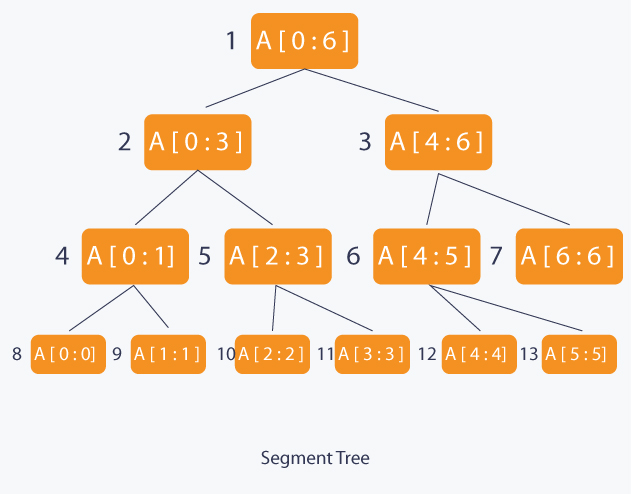# 线段树# 线段树合并分裂

Segment merge(Segment a, Segment b){
if(a == NIL) return b;
if(b == NIL) return a;
//递归合并线段树a、b
}


# 线段树上的搜索问题

1. 给定$l,r,x$，对于$l\leq i\leq r$，将$a_i$修改为$a_i+x$。
2. 给定$l,r,x$，查询所有满足$l\leq i\leq r$且$a_i\geq x$的下标$i$中最小的那个下标，如果不存在，则输出$-1$，否则输出下标

1. 顶点所代表的区间完全落在查询区间中
2. 顶点所代表的区间部分落在查询区间中
3. 顶点所代表的区间与查询区间无交点

1. 给定$l,r,x$，对于$l\leq i\leq r$，将$a_i$修改为$a_i+x$。
2. 给定$l,r,x$，查询所有满足$l\leq j < i \leq r$且$a_i+a_j\geq x$的下标$i$中最小的那个下标，如果不存在，则输出$-1$，否则输出下标

# Segment tree beats

1. 给定$l,r,x$，对于区间$l\leq i\leq r$，将$a_i$替换为$\min(a_i,x)$。
2. 给定$l,r$，查询$\max(a_l,a_{l+1},\ldots, a_r)$。
3. 给定$l,r$，查询$a_l+a_{l+1}+\ldots+a_r$。

1. 如果当前区间中最大值小于等于$x$，那么更新没有意义，直接返回即可。
2. 如果当前区间中最大值大于$x$，但是次大值小于$x$，那么我们就打上更新标记，同时修改区间和（由于最大值出现次数也在维护，因此新的区间和是可以直接计算的）。
3. 如果当前区间中次大值大于等于$x$（当次大值等于$x$时，这时候虽然我们能成功更新区间和，但是严格次大值就是非严格的了）。因此我们这时候必须暴力更新左右子区间，再利用左右子区间更新后的信息更新当前区间的信息。

1. 给定$l,r,x$，对于区间$l\leq i\leq r$，将$a_i$替换为$\min(a_i,x)$。
2. 给定$l,r,x$，对于区间$l\leq i\leq r$，将$a_i$替换为$a_i+x$。
3. 给定$l,r$，查询$\max(a_l,a_{l+1},\ldots, a_r)$。
4. 给定$l,r$，查询$a_l+a_{l+1}+\ldots+a_r$。

1. 给定$l,r,x$，对于区间$l\leq i\leq r$，将$a_i$替换为$\min(a_i,x)$。
2. 给定$l,r,x$，对于区间$l\leq i\leq r$，将$a_i$替换为$\max(a_i,x)$。
3. 给定$l,r$，查询$\max(a_l,a_{l+1},\ldots, a_r)$。
4. 给定$l,r$，查询$\min(a_l,a_{l+1},\ldots, a_r)$。
5. 给定$l,r$，查询$a_l+a_{l+1}+\ldots+a_r$。